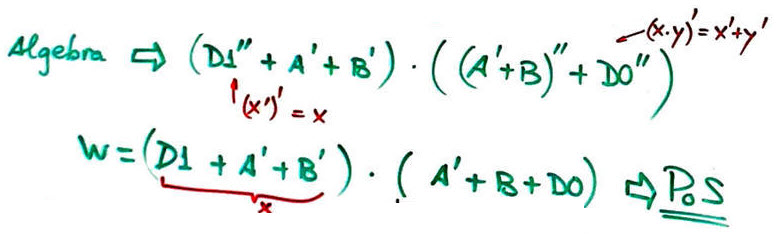﻿ Digital Circuits and Systems - Circuits i Sistemes Digitals (CSD) - EETAC - UPCBachelor's Degree in Telecommunications Systems and in Network EngineeringLecture 3 L1.3: Circuit analysis using Boole's algebra (method III) P1 analysis. Example Circuit_W (a section of Circuit_C) [23/2]

1.4. Analysis concept map: several methods for analysing simple combinational circuitsFig. 1. Analysisi map proposing several methods to deduce the circuit's truth table.

1.4.1. Method I: Proteus simulation for truth tables and deducing logic circuits (Lab1_1)

1.4.2. Method II: WolframAlpha engine for calculating truth tables and deducing logic circuits (Lab1_1)

1.4.3. Method III: Pen & paper analysis using Boolean algebra

1.4.3.1. Properties of boolean functions

1.4.3.2. Duality principle, De Morgan's laws

1.4.3.3. Product of sums (PoS)

1.4.3.4. Sum of products (SoP)

1.4.3.5. Any type of equation or non-standard forms

To continue with the example circuit, to solve step 2, it is better to conceive it as a project:

Specifications: Analyse a section of Circuit_C proposed in to be named Circuit_W represented in Fig.2. Find its truth table using method III. This is a on the idea of circuit analysis.Fig. 2. Circuit_W symbol and internal architecture.

Planning: follow our concept map in Fig. 3Fig. 3. Plan for analysis method III.
• Firstly, we deduce the circuit's equation: the logic diagram translated to an algebraic equation.

• Secondly, applying algebra we simplify the circuit equation to obtain PoS.

• Thirdly, we add missing variables to convert sums into maxterms using the identity x = (x+y)·(x+y'), and the product of maxterms is already the solution.

This is the folder where to save your notes, pictures, scanned diagrams and discussion:

C:\CSD\P1\Lab1_1\Circuit_W\Algebra\(files)

Development. This is Circuit_W solution using the proposed analysis method III.Fig. 3. Developing the project using algebra.

Alternatively, from the circuit equation we obtain a SoP that becomes the sum of minterms adding missing variables in each product using x = x·(x+y').

Testing: This time, testing the validity of the truth table will be simply comparing solutions from other methods.

To complete step 3, repeat and apply all these concepts to Circuit_C proposed in

1.4.4. Method IV: Analysis using synthesis and simulation EDA tools (Lab1_2)